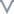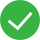# 字符函数

### 示例

``````UPPER([客户名称]) = JANE JOHNSON
``````

### 平台中的数值函数

CONTAINS - 如果给定字符串包含指定子字符串，则返回 true。

ENDSWITH - 如果给定字符串以指定子字符串结尾，则返回 true。

FIND - 返回字符串中子字符串的索引位置，如果未找到子字符串，则返回 0。

FINDNTH - 返回指定字符串内的第 n 个子字符串的位置，其中 n 由 occurrence 参数定义。

LEFT - 返回字符串最左侧一定数量的字符。

LEN - 返回字符串的字符长度。

LOWER - 返回字符串，其所有字符为小写。

LTRIM - 返回移除了所有前导空格的字符串。

MAX - 对于字符串，MAX 查找数据库为该列定义的排序序列中的最高值。

MID - 返回从索引位置开始的字符串。字符串中第一个字符的位置为 1。如果添加了可选参数 length，则返回的字符串仅包含该数量的字符。

MIN - 对于字符串，MIN 查找排序序列中的最低值。

REPLACE - 在字符串中搜索子字符串，并将其替换为替代子字符串。

RIGHT - 返回字符串最右侧一定数量的字符。

RTRIM - 返回移除了所有尾随空格的字符串。

SPACE - 返回由指定数量的重复空格组成的字符串。

SPLIT - 返回字符串中的一个子字符串，并使用分隔符字符将字符串分为一系列标记。

STARTSWITH - 如果字符串以子字符串开头，则返回 true。会忽略前导空格。

TRIM - 返回移除了前导和尾随空格的字符串。

UPPER - 返回字符串，其所有字符为大写。

## 转为字符串:STR(表达式)

### 示例

STR(200) = “200” ，返回是字符串类型

## 截取字符串:SUBSTR(字符串，开始位置，[长度])

### 示例

SUBSTR(“abc123”, 1, 3) = “abc”， SUBSTR(“abc123”, 1) = “abc123”

## 字符串长度:LENGTH(字符串)

### 示例

LENGTH(“abc123”) = 6

## 替换字符串:REPLACE(字符串，子串，替换串)

### 示例

REPLACE(“Calculation”, “ion”, “ed”) = “Calculated”

## 字符串转大写:UPPER(字符串)

### 示例

UPPER(“product”) = “PRODUCT”

## 字符串转小写:LOWER(字符串)

### 示例

LOWER(“PRODUCT”) = “product”

## 移除前导空格:LTRIM(字符串)

### 示例

LTRIM(" Sales") = “Sales”

## 移除尾随空格:RTRIM(字符串)

### 示例

RTRIM("Market ") = “Market”

## JSON字段提取:JSON_EXTRACT(字段访问器, JSON字符串)

### 示例

• JSON_EXTRACT("", “[1,2,[3,4]]”) = “4”
• JSON_EXTRACT("", “[“a”,“b”]”) = “b”
• JSON_EXTRACT(“xy”, “{“xy”:1, “y”:2}”) = “1”
• JSON_EXTRACT(".x.z", “{“x”:{“z”:1}, “y”:2}”) = “1”
• JSON_EXTRACT(“x”, “{“x”:{“z”:1}, “y”:2}”) = “{ z: 1}”
• JSON_EXTRACT(".x.y", “{“x”: [1,{“y”:2.0}], “z”:3.0}”) = “2”
• JSON_EXTRACT(".x.y", “{“x”: [1,{“y”:2.0}], “z”:3.0}”) = “2”

## 包含:CONTAINS(字符串, 子串)

### 示例

• CONTAINS(“Calculation”, “alcu”) = true
• CONTAINS(“Calculation”, “beu”) = false

## 结尾包含:ENDWITH(字符串, 子串)

### 示例

• ENDSWITH(“Calculation”, “tion”) = true

## 开头包含:STARTWITH(字符串, 子串)

### 示例

• STARTSWITH(“Calculation”, “Ca”) = true

## 开头截取:LEFT(字符串, 整数)

### 示例

• LEFT(“Calculation”, 4) = “Calc”

## 结尾截取:RIGHT(字符串, 整数)

### 示例

• RIGHT(“Calculation”, 4) = “tion”

## 查找字符串位置:FIND(字符串, 子串, [起始位置]))

### 示例

• FIND(“Calculation”, “alcu”) = 2

## 正则捕获提取:REGEXP_EXTRACT_NTH(表达式, 模式, 索引)

### 示例

• EGEXP_EXTRACT_NTH(“abc 123”, “([a-z])+\s+(\d+)”, 2) = “123”

## 正则替换:REGEXP_REPLACE(字符串, 模式, 被替换串)

### 示例

• REGEXP_REPLACE(“abc 123”, “\s”, “-”) = “abc–123”根本没帮助文档较差文档一般文档不错文档很好

#### 更多建议

0/200